# Chapter 6 The Elasticity of Demand The Concept

• Slides: 32
Download presentation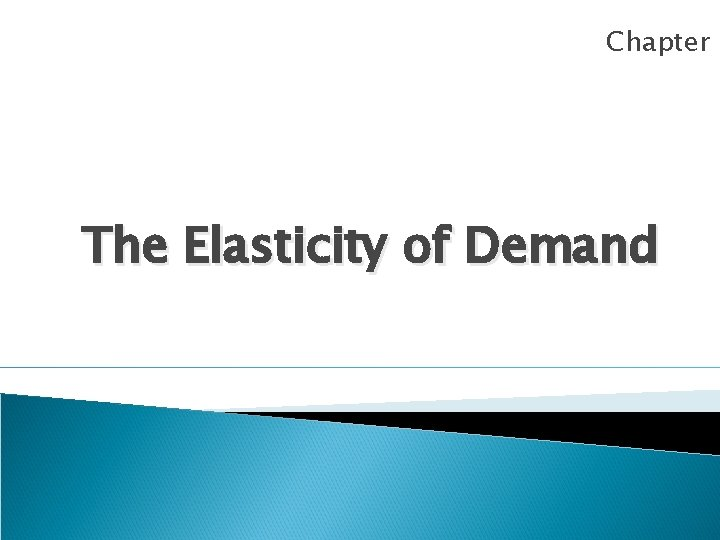Chapter 6 The Elasticity of Demand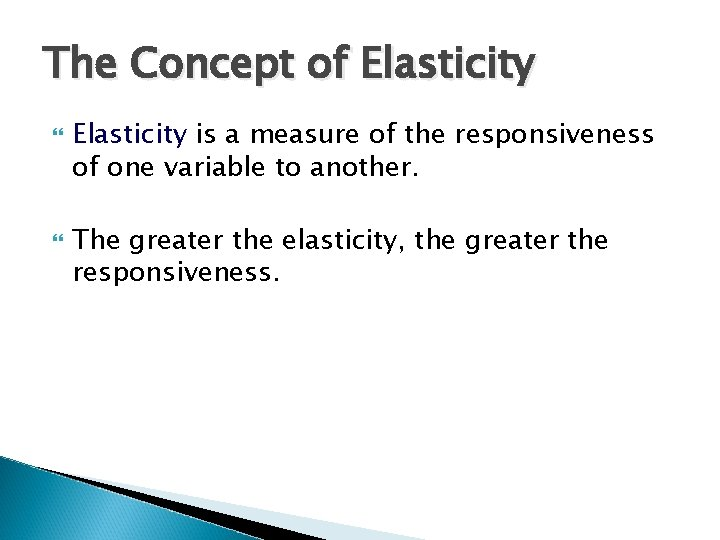The Concept of Elasticity is a measure of the responsiveness of one variable to another. The greater the elasticity, the greater the responsiveness.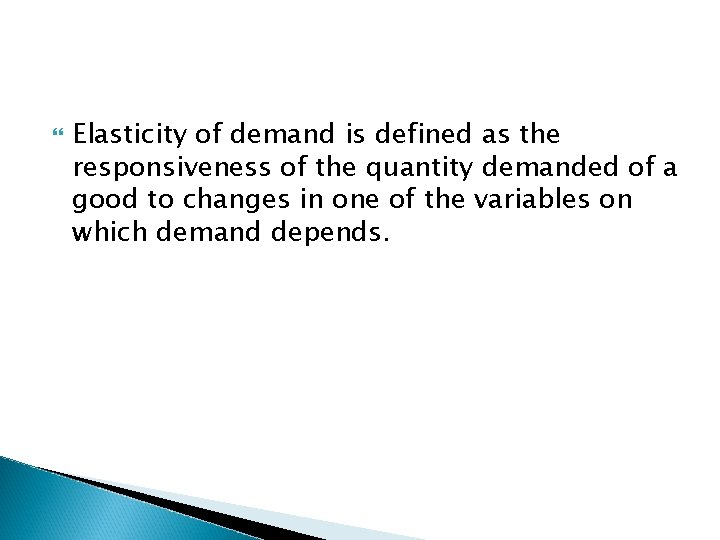Elasticity of demand is defined as the responsiveness of the quantity demanded of a good to changes in one of the variables on which demand depends.These variables are price of the commodity, prices of the related commodities, income of the consumer & other various factors on which demand depends. Thus, we have Price Elasticity, Cross Elasticity, Elasticity of Substitution & Income Elasticity. It is always price elasticity of demand which is referred to as elasticity of demand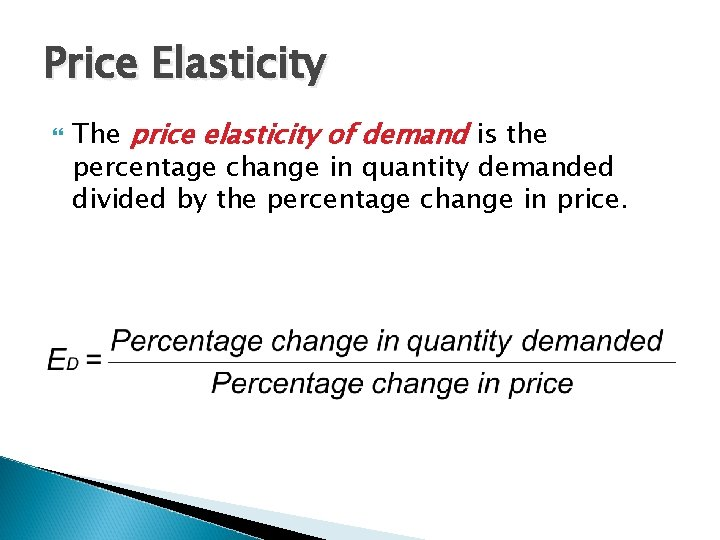Price Elasticity The price elasticity of demand is the percentage change in quantity demanded divided by the percentage change in price.Price Elasticity ep = Percentage Change in Quantity Demanded Percentage Change in Price = Change in Quantity Demanded Initially = Change in Price Initial Price P ∆Qd ∆P ÷ * Qd Where ∆Qd = Change in Quantity Demanded ∆P = Change in Price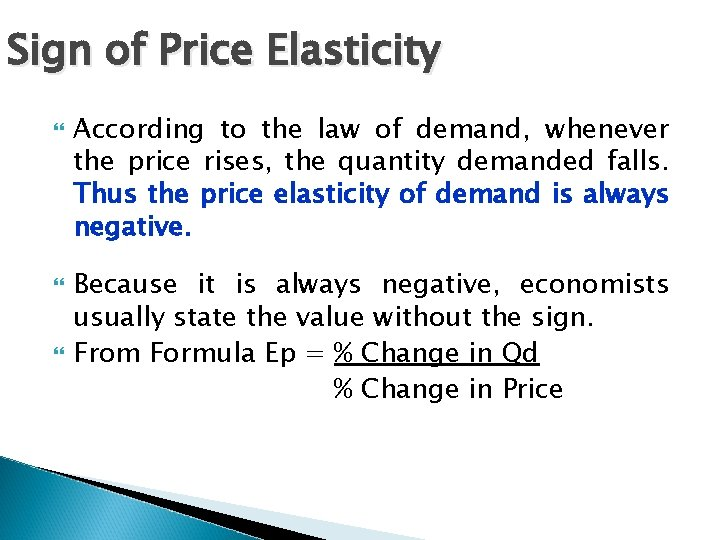Sign of Price Elasticity According to the law of demand, whenever the price rises, the quantity demanded falls. Thus the price elasticity of demand is always negative. Because it is always negative, economists usually state the value without the sign. From Formula Ep = % Change in Qd % Change in PricePrice Elasticity of Demand If Price Elasticity of Demand > 1, demand is elastic, When quantity demanded responds greatly to price changes If Price Elasticity of Demand = 1, demand is unit elastic, When quantity demanded responds equally to price changes. If Price Elasticity of Demand < 1, demand is inelastic , When quantity demanded responds little to price changes. Perfectly inelastic Perfectly elastic or infinite elastic demandTypes of Price Elasticity Type Perfectly elastic Numerical Description Shape of expression curve ∞ Infinite Horizontal Perfectly 0 inelastic Unit elastic 1 Relatively elastic Relatively inelastic Zero Vertical One >1 Rectangular hyperbola More than 1 Flat <1 Less than 1 Steep 9Types of Price Elasticity Ep=0 price Ep= ∞ p r i c e Ep> 1 Ep=1 Ep<1 Quantity demanded 10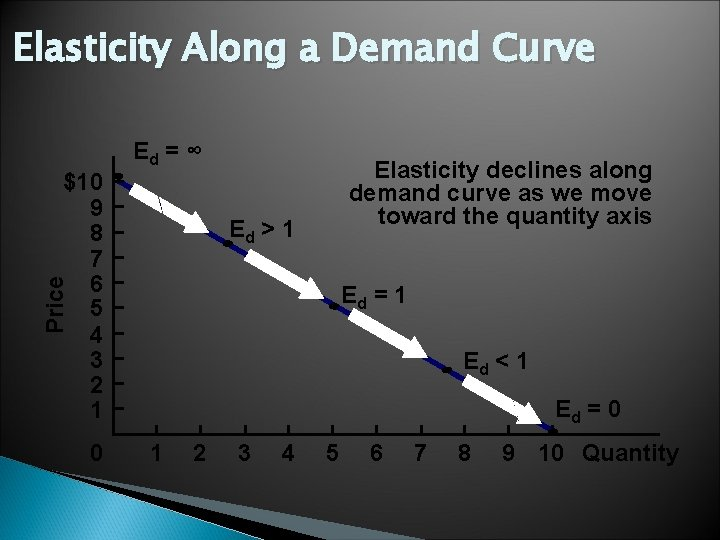Elasticity Along a Demand Curve Ed = ∞ \$10 9 8 7 6 5 4 3 2 1 Elasticity declines along demand curve as we move toward the quantity axis Price Ed > 1 0 Ed = 1 Ed < 1 Ed = 0 1 2 3 4 5 6 7 8 9 10 Quantity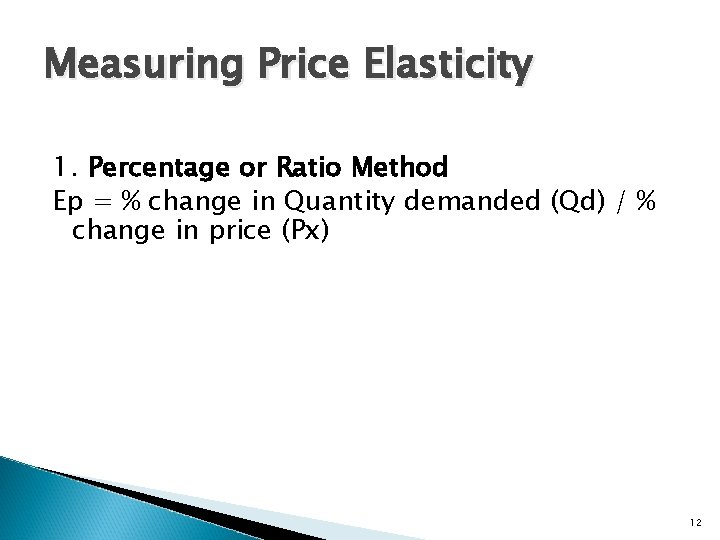Measuring Price Elasticity 1. Percentage or Ratio Method Ep = % change in Quantity demanded (Qd) / % change in price (Px) 12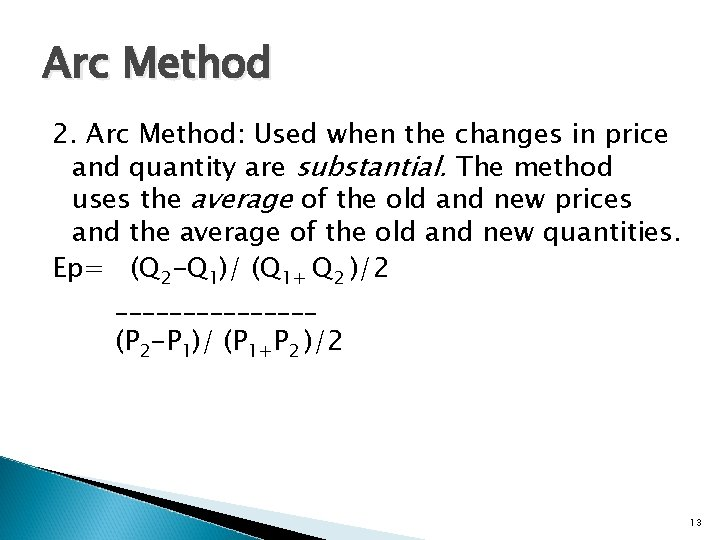Arc Method 2. Arc Method: Used when the changes in price and quantity are substantial. The method uses the average of the old and new prices and the average of the old and new quantities. Ep= (Q 2 -Q 1)/ (Q 1+ Q 2 )/2 ________ (P 2 -P 1)/ (P 1+P 2 )/2 13Total Outlay Method 3. Total Outlay Method Elasticity is measured by comparing total expenditure before and after a change in price Ep=1: Unit elastic when there is no change in the total revenue as a result of a rise or fall in price - Revenue remains constant Ep>1: Relatively elastic when total revenue rises with a fall in price and falls with a rise in price, Ep<1 : Relatively inelastic when total revenue rises with a rise in price and falls with a fall in price, 14Revenue Concepts Total Revenue: TR = P * Q Average Revenue AR = TR/Q = (P*Q)/Q = P Marginal Revenue MRn = TRn – TRn-1 OR MRn = d(TR)/d. QTotal Outlay Method Elasticity Price Quantity demanded Total Outlay( TR) Highly elastic ( Ep >1) Increases Decreases Increases Decreases No Change Decreases Increases No Change Increases Decreases Unitary Elastic ( Ep=1) Highly inelastic (Ep < 1) 16Total Outlay Method Unit Price (Rs) A. B. C. Quantity TE =P*Q (Rs) 10 10 100 5 25 10 10 100 5 20 10 10 100 5 15 75 ep>1 ep=1 ep<1 17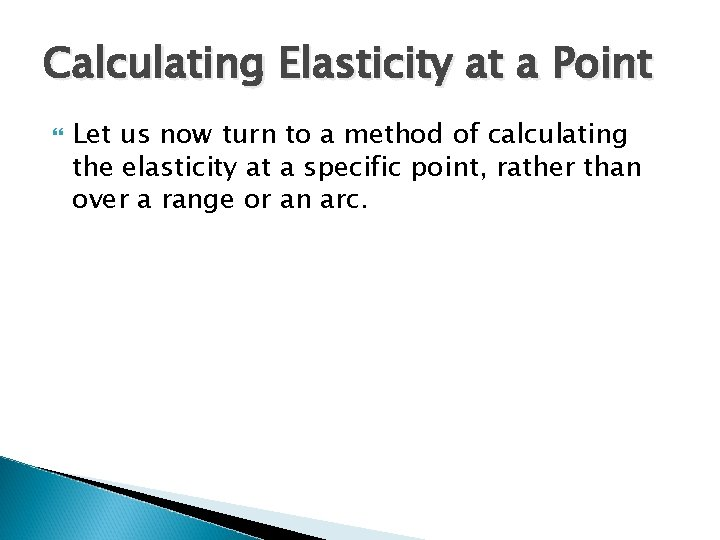Calculating Elasticity at a Point Let us now turn to a method of calculating the elasticity at a specific point, rather than over a range or an arc.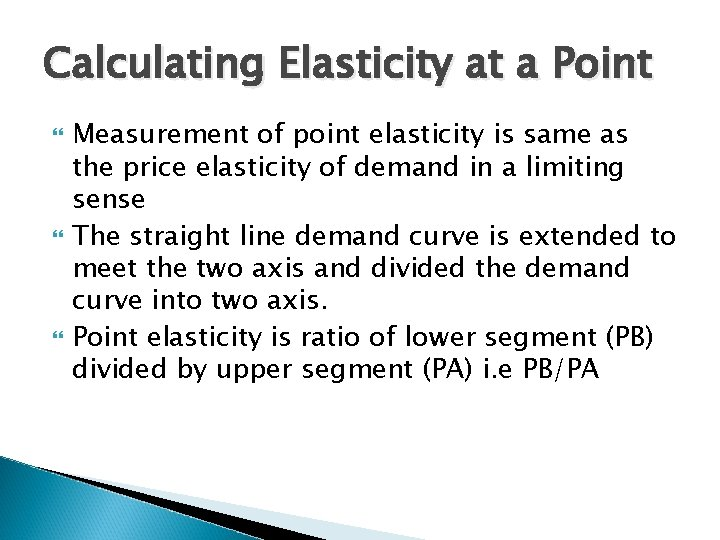Calculating Elasticity at a Point Measurement of point elasticity is same as the price elasticity of demand in a limiting sense The straight line demand curve is extended to meet the two axis and divided the demand curve into two axis. Point elasticity is ratio of lower segment (PB) divided by upper segment (PA) i. e PB/PACalculating Elasticity at a Point Ø Slope of the Demand Curve d. Q d. P Ø Point Elasticity on the demand curve ep = d. Q P d. P Q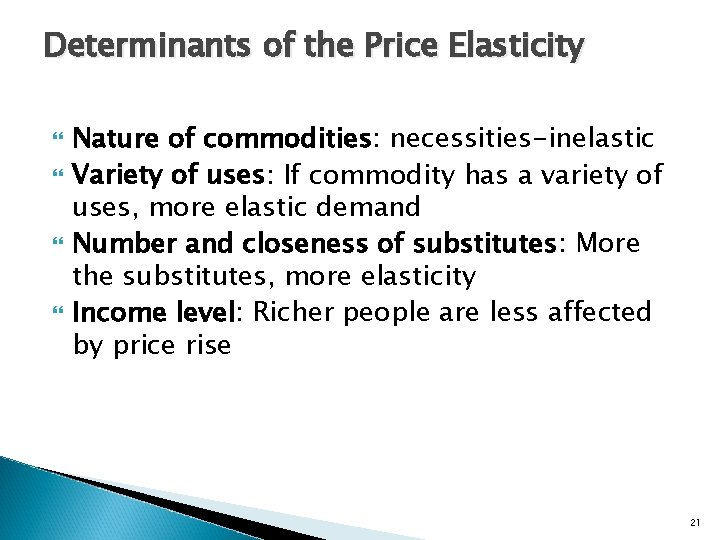Determinants of the Price Elasticity Nature of commodities: necessities-inelastic Variety of uses: If commodity has a variety of uses, more elastic demand Number and closeness of substitutes: More the substitutes, more elasticity Income level: Richer people are less affected by price rise 21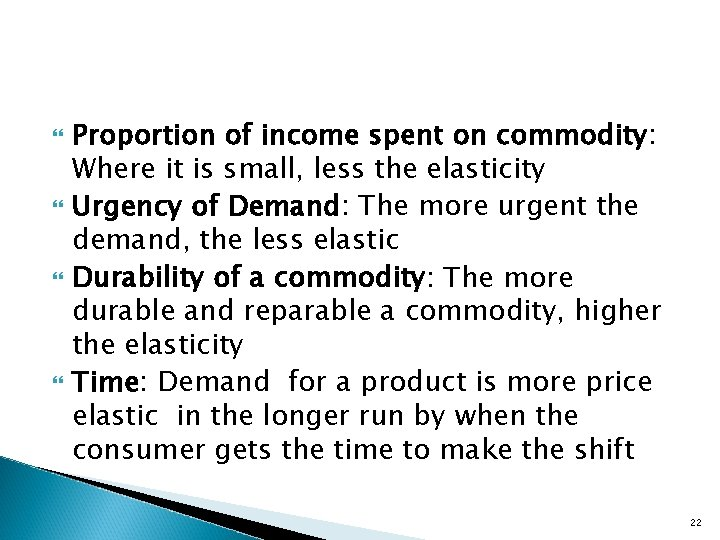Proportion of income spent on commodity: Where it is small, less the elasticity Urgency of Demand: The more urgent the demand, the less elastic Durability of a commodity: The more durable and reparable a commodity, higher the elasticity Time: Demand for a product is more price elastic in the longer run by when the consumer gets the time to make the shift 22Marginal Revenue Marginal revenue (MR) is the change in total revenue per unit change in output Since MR measures the rate of change in total revenue as quantity changes, MR is the slope of the total revenue (TR) curve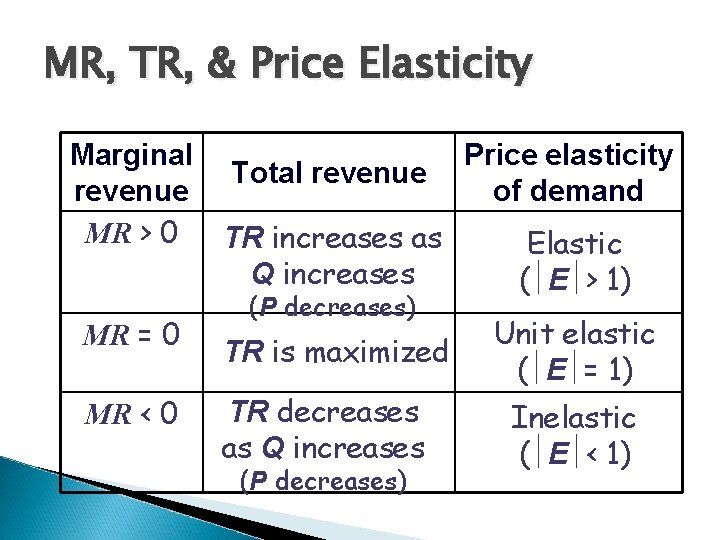MR, TR, & Price Elasticity Marginal revenue MR > 0 MR = 0 MR < 0 Price elasticity Total revenue of demand TR increases as Elastic ( E > 1) 1) Q increases ( E > (P decreases) Unit elastic TR is maximized ( E = 1) 1) TR decreases Inelastic as Q increases ( E < 1) 1) ( E < (P decreases)Marginal Revenue & Price Elasticity For all demand & marginal revenue curves, the relation between marginal revenue, price, & elasticity can be expressed asRelationship between ep , AR, MR ep = d. Q P d. P Q MR = d TR d. Q MR = d P. Q d. Q MR = P { 1 + Q d. P } P d. Q AR = P (Price) TR = P. Q MR = P + Q. d. P d. Q MR = AR { 1 + 1} Ep The difference between AR and MR depends inversely on the absolute value of the elasticity of demand.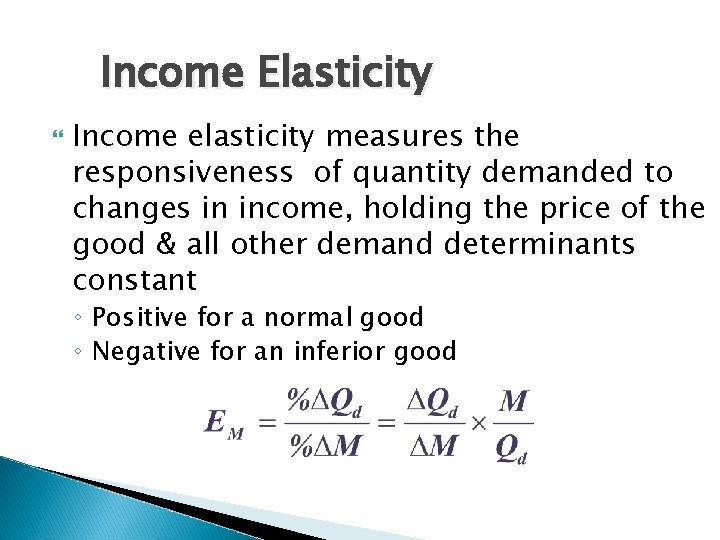Income Elasticity Income elasticity measures the responsiveness of quantity demanded to changes in income, holding the price of the good & all other demand determinants constant ◦ Positive for a normal good ◦ Negative for an inferior goodIncome Elasticity E 1 Q 2 Income elasticity of demand is greater than one. In this case as income increases, the quantity demanded also increases. But the percentage increase in demand is more than percentage increase in income Quantity Demanded Q 1 0 E Y 1 Y 2 Income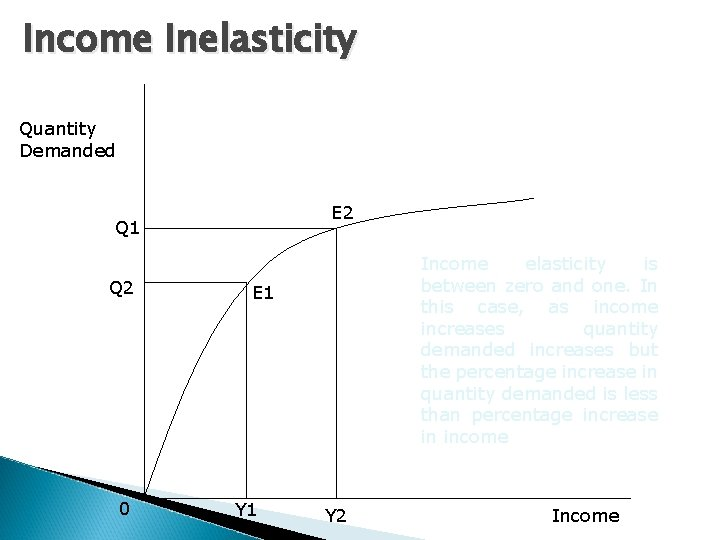Income Inelasticity Quantity Demanded E 2 Q 1 Q 2 0 Income elasticity is between zero and one. In this case, as income increases quantity demanded increases but the percentage increase in quantity demanded is less than percentage increase in income E 1 Y 2 IncomeIncome Elasticity EM 1 0< <0 EM ED Example Income Elastic Luxury Products Income Inelastic Staple Products Negative Elastic Inferior ProductsCross-Price Elasticity Cross-price elasticity (EXY) measures the responsiveness of quantity demanded of good X to changes in the price of related good Y, holding the price of good X & all other demand determinants for good X constant ◦ Positive when the two goods are substitutes ◦ Negative when the two goods are complements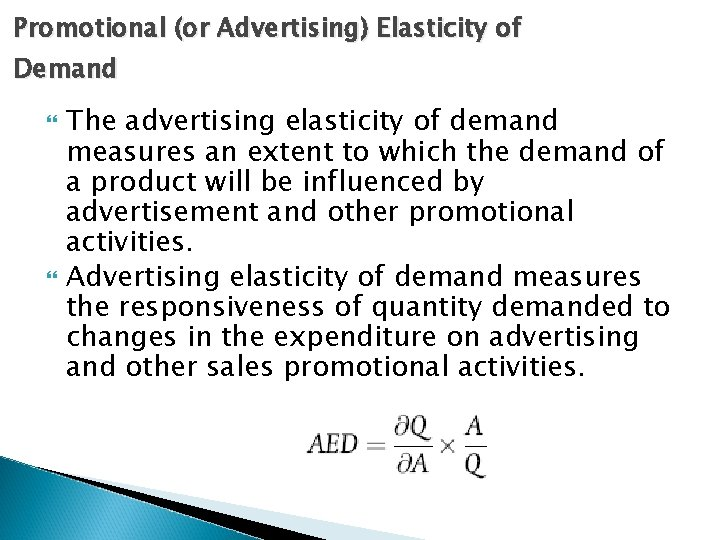Promotional (or Advertising) Elasticity of Demand The advertising elasticity of demand measures an extent to which the demand of a product will be influenced by advertisement and other promotional activities. Advertising elasticity of demand measures the responsiveness of quantity demanded to changes in the expenditure on advertising and other sales promotional activities.